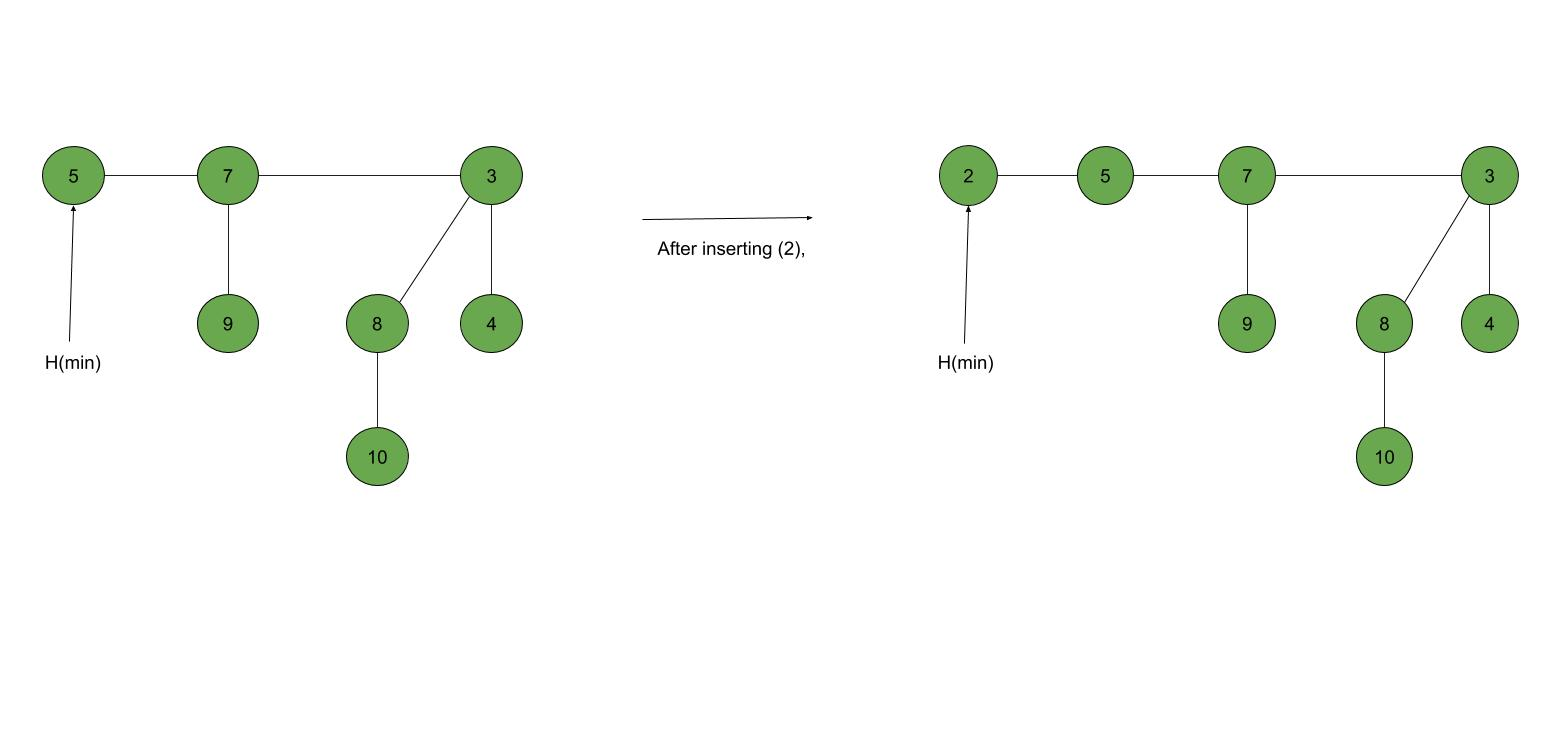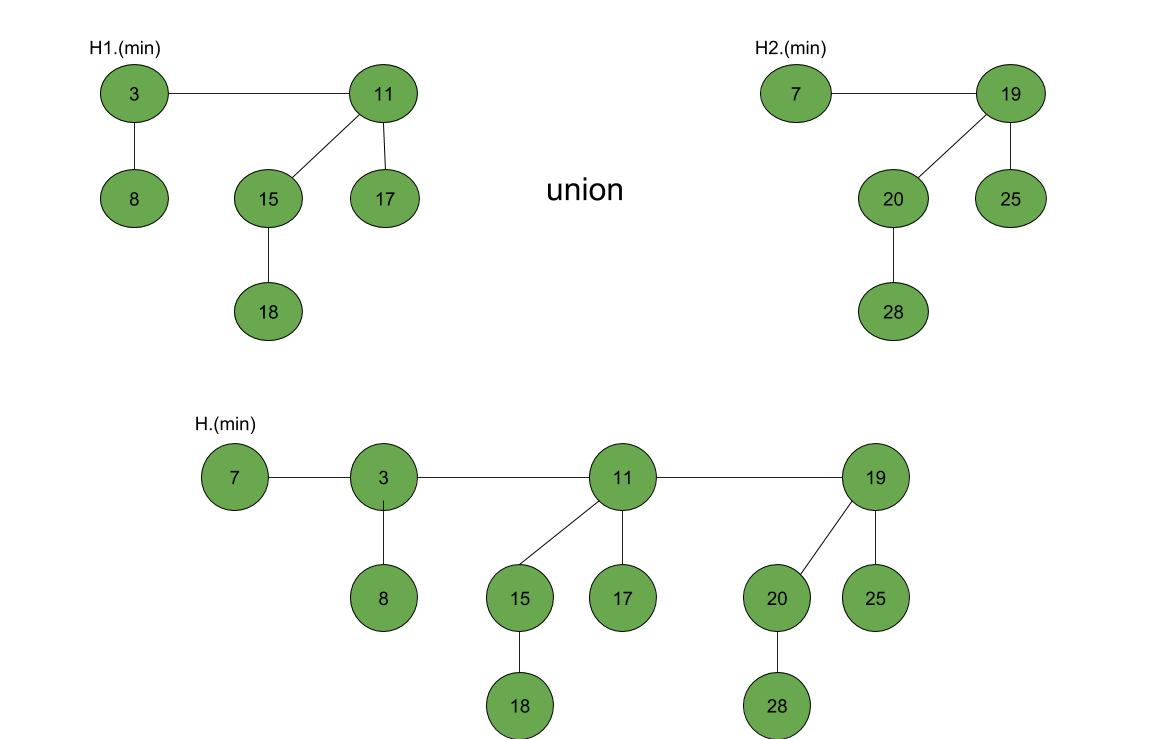Related Articles

# Fibonacci Heap – Insertion and Union

• Difficulty Level : Medium
• Last Updated : 14 Dec, 2020

Prerequisites: Fibonacci Heap (Introduction)

Fibonacci Heap is a collection of trees with min-heap or max-heap property. In Fibonacci Heap, trees can have any shape even all trees can be single nodes (This is unlike Binomial Heap where every tree has to be Binomial Tree).
In this article, we will discuss Insertion and Union operation on Fibonacci Heap.

Insertion: To insert a node in a Fibonacci heap H, the following algorithm is followed:

1. Create a new node ‘x’.
2. Check whether heap H is empty or not.
3. If H is empty then:
• Make x as the only node in the root list.
• Set H(min) pointer to x.
4. Else:
• Insert x into root list and update H(min).

Example:Union: Union of two Fibonacci heaps H1 and H2 can be accomplished as follows:

1. Join root lists of Fibonacci heaps H1 and H2 and make a single Fibonacci heap H.
2. If H1(min) < H2(min) then:
• H(min) = H1(min).
3. Else:
• H(min) = H2(min).

Example:Following is a program to demonstrate building and inserting in a Fibonacci heap:

## C++

 `// C++ program to demonstrate building` `// and inserting in a Fibonacci heap` `#include ` `#include ` `#include ` `using` `namespace` `std;`   `struct` `node {` `    ``node* parent;` `    ``node* child;` `    ``node* left;` `    ``node* right;` `    ``int` `key;` `};`   `// Creating min pointer as "mini"` `struct` `node* mini = NULL;`   `// Declare an integer for number of nodes in the heap` `int` `no_of_nodes = 0;`   `// Function to insert a node in heap` `void` `insertion(``int` `val)` `{` `    ``struct` `node* new_node = (``struct` `node*)``malloc``(``sizeof``(``struct` `node));` `    ``new_node->key = val;` `    ``new_node->parent = NULL;` `    ``new_node->child = NULL;` `    ``new_node->left = new_node;` `    ``new_node->right = new_node;` `    ``if` `(mini != NULL) {` `        ``(mini->left)->right = new_node;` `        ``new_node->right = mini;` `        ``new_node->left = mini->left;` `        ``mini->left = new_node;` `        ``if` `(new_node->key < mini->key)` `            ``mini = new_node;` `    ``}` `    ``else` `{` `        ``mini = new_node;` `    ``}` `}`   `// Function to display the heap` `void` `display(``struct` `node* mini)` `{` `    ``node* ptr = mini;` `    ``if` `(ptr == NULL)` `        ``cout << ``"The Heap is Empty"` `<< endl;`   `    ``else` `{` `        ``cout << ``"The root nodes of Heap are: "` `<< endl;` `        ``do` `{` `            ``cout << ptr->key;` `            ``ptr = ptr->right;` `            ``if` `(ptr != mini) {` `                ``cout << ``"-->"``;` `            ``}` `        ``} ``while` `(ptr != mini && ptr->right != NULL);` `        ``cout << endl` `             ``<< ``"The heap has "` `<< no_of_nodes << ``" nodes"` `<< endl;` `    ``}` `}` `// Function to find min node in the heap` `void` `find_min(``struct` `node* mini)` `{` `    ``cout << ``"min of heap is: "` `<< mini->key << endl;` `}`     `// Driver code` `int` `main()` `{`   `    ``no_of_nodes = 7;` `    ``insertion(4);` `    ``insertion(3);` `    ``insertion(7);` `    ``insertion(5);` `    ``insertion(2);` `    ``insertion(1);` `    ``insertion(10);`   `    ``display(mini);`   `    ``find_min(mini);`   `    ``return` `0;` `}`

Output:

```The root nodes of Heap are:
1-->2-->3-->4-->7-->5-->10
The heap has 7 nodes
Min of heap is: 1```

Attention reader! Don’t stop learning now. Get hold of all the important DSA concepts with the DSA Self Paced Course at a student-friendly price and become industry ready.  To complete your preparation from learning a language to DS Algo and many more,  please refer Complete Interview Preparation Course.

In case you wish to attend live classes with experts, please refer DSA Live Classes for Working Professionals and Competitive Programming Live for Students.

My Personal Notes arrow_drop_up
Recommended Articles
Page :